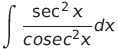Select Page

# CBSE Application of Integrals Maths 12 Science Answers for MCQ in English

CBSE Application of Integrals Maths 12 Science Answers for MCQ in English to enable students to get Answers in a narrative video format for the specific question.

Expert Teacher provides CBSE Application of Integrals Maths 12 Science Answers for MCQ through Video Answers in English language. This video solution will be useful for students to understand how to write an answer in exam in order to score more marks. This teacher uses a narrative style for a question from Application of Integrals not only to explain the proper method of answering question, but deriving right answer too.

Please find the question below and view the Answer in a narrative video format.

Question:

## Similar Questions from CBSE, 12th Science, Maths, Application of Integrals

Question 1 : Find the area of smaller region bounded by the ellipseand the line. (View Answer Video)

Question 2 :

Find the area of the given curves and given lines:and x-axis

Question 3 : Find the area bounded by curvesand(View Answer Video)

Question 4 : Find the area of the region bounded by the curveand the line x = 3. (View Answer Video)

Question 5 : Using the method of integration, find the area of the region bounded by the lines 3x - y - 3 = 0, 2x + y - 12 = 0 and x -2y - 1 = 0. (View Answer Video)

### Integrals

Question 1 : Evaluate the following :(View Answer Video)

Question 2 : Evaluate :. (View Answer Video)

Question 3 : Find the integral of the function. (View Answer Video)

Question 4 :(View Answer Video)

Question 5 : Write the value of:(View Answer Video)

### Continuity and Differentiability

Question 1 : Differentiate w.r.t.x the function, for some fixed a > 0 and x > 0. (View Answer Video)

Question 2 : Differentiate w.r.t.x the function. (View Answer Video)

Question 3 : Findfor the function. (View Answer Video)

Question 4 :  Find the second order derivative of the function. (View Answer Video)

Question 5 : Findfor the function. (View Answer Video)

### Determinant

Question 1 : Iffind |A|. (View Answer Video)

Question 2 : If a, b, c are in A.P., then the determinantis (View Answer Video)

Question 4 :  Find the values of x, if. (View Answer Video)
Question 5 : Using the properties of determinants, evaluate. (View Answer Video)